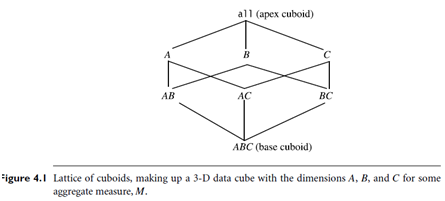Introduction: Data generalization is a process that abstracts a large set of task-relevant data in a database from a relatively low conceptual level to higher conceptual levels. Users like the ease and flexibility of having large data sets summarized in concise and succinct terms, at different levels of granularity, and from different angles. Such data descriptions help provide an overall picture of the data at hand.

Efficient Methods for Data Cube Computation

Data cube computation is an essential task in data warehouse implementation. The pre computation of all or part of a data cube can greatly reduce the response time and enhance the performance of on-line analytical processing. However, such computation is challenging because it may require substantial computational time and storage space. This section explores efficient methods for data cube computation.

A Road Map for the Materialization of Different Kinds of Cubes

Data cubes facilitate the on-line analytical processing of multidimensional data. For completeness, we begin with a review of the basic terminology involving data cubes. We also introduce a cube cell notation that is useful for describing data cube computation methods.Cube Materialization: Full Cube, Iceberg Cube, Closed Cube, and Shell Cube

Figure 4.1 shows a 3-D data cube for the dimensions A, B, and C, and an aggregate measure, M. A data cube is a lattice of cuboids. Each cuboid represents a group-by. ABC is the base cuboid, containing all three of the dimensions. Here, the aggregate measure, M, is computed for each possible combination of the three dimensions. The base cuboid is the least generalized of all of the cuboids in the data cube. The most generalized cuboid is the apex cuboid, commonly represented as all. It contains one value—it aggregates measure M for all of the tuples stored in the base cuboid. To drill down in the data cube, we move from the apex cuboid, downward in the lattice. To roll up, we move from the base cuboid, upward. For the purposes of our discussion in this chapter, we will always use the term data cube to refer to a lattice of cuboids rather than an individual cuboid.

A cell in the base cuboid is a base cell. A cell from a non base cuboid is an aggregate cell. An aggregate cell aggregates over one or more dimensions, where each aggregated dimension is indicated by a “

” in the cell notation. Suppose we have an n-dimensional data cube. Let a = (a1, a2, : : : , an, measures) be a cell from one of the cuboids making up the data cube.We say that a is an m-dimensional cell (that is, from an m-dimensional cuboid) if exactly m (m ≤ n) values among fa1, a2, : : : , ang are not “ * ”. If m = n, then a is a base cell; otherwise, it is an aggregate cell (i.e., where m < n).

General Strategies for Cube Computation: With different kinds of cubes as described above, we can expect that there are a good number of methods for efficient computation. In general, there are two basic data structures used for storing cuboids. Relational tables are used as the basic data structure for the implementation of relational OLAP (ROLAP), while multidimensional arrays are used as the basic data structure in multidimensional OLAP (MOLAP). Although ROLAP and MOLAP may each explore different cube computation techniques, some optimization “tricks” can be shared among the different data representations. The following are general optimization techniques for the efficient computation of data cubes.

Optimization Technique 1: Sorting, hashing, and grouping. Sorting, hashing, and grouping operations should be applied to the dimension attributes in order to reorder and cluster related tuples.

In cube computation, aggregation is performed on the tuples (or cells) that share the same set of dimension values. Thus it is important to explore sorting, hashing, and grouping operations to access and group such data together to facilitate computation of such aggregates. For example, to compute total sales by branch, day, and item, it is more efficient to sort tuples or cells by branch, and then by day, and then group them according to the item name. Efficient implementations of such operations in large data sets have been extensively studied in the database research community. Such implementations can be extended to data cube computation.